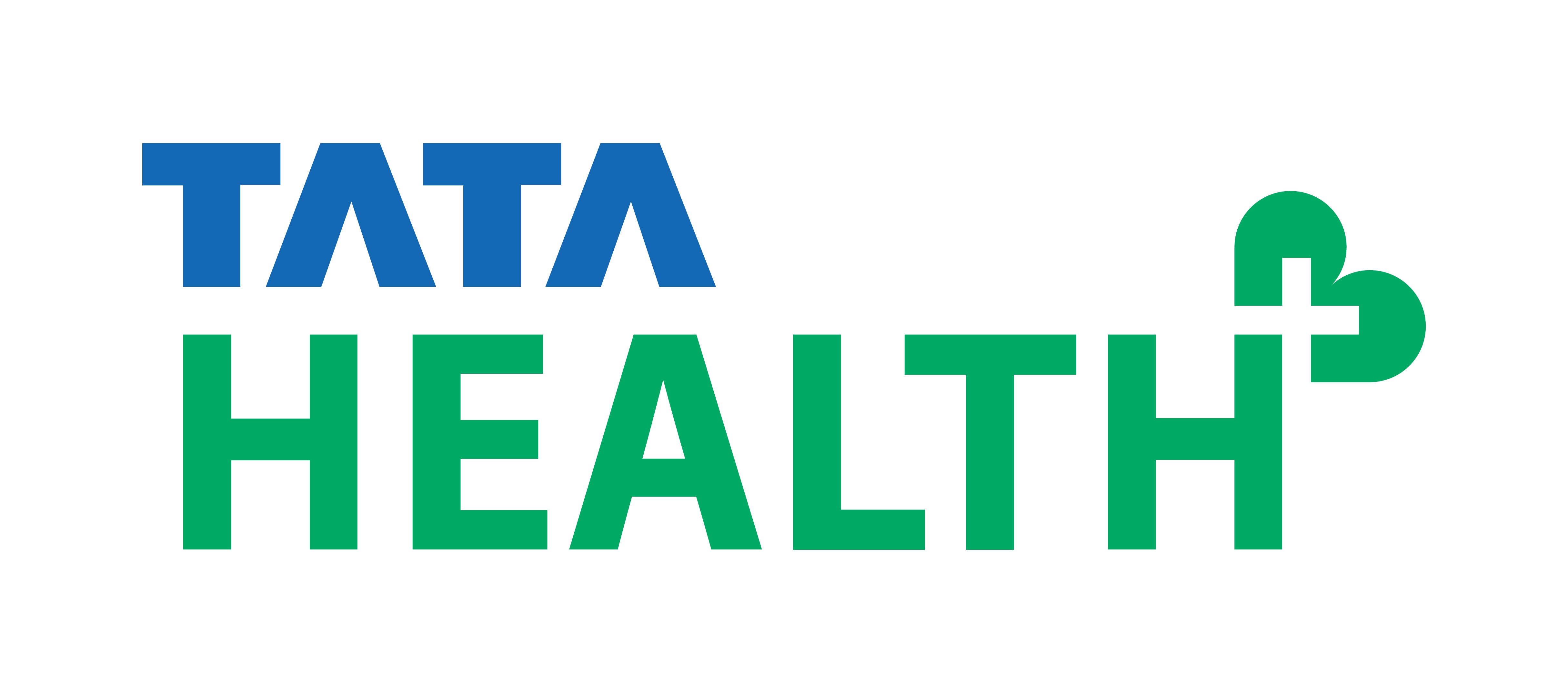Maximize Array Sum
Tag(s):

## Algorithms, Easy, Greedy algorithm

Problem
Editorial
Analytics

You are given an array  $A$ of size $N$. You can fix any element, say $K$ of the array and all the elements in the array greater than or equal to $K$ become $K$ and all the elements less than $K$ multiplied by -1. You have to find the maximum sum of the array after fixing $K$ .

Input Format

The first line contains T, the number of test cases.
For each Test case :
The first line contains an integer N, size of the array.
The second line contains N space-separated integers, the $i^{th}$ of which is $A[i]$.

Input Constraints

$1 \le T \le 10^{ 5}$
$2 \le N \le 10^{5}$
$0 \le A[i] \le 10^{6}$
Sum of N all over testcases doesn't not exceed $10^{6}$.

Output Format

For each test case, print the maximum sum of the array by fixing an element $K$.
Answer for each test case should come in a new line.

Note

Candidates are expected to write complete code of searching, sorting etc. (if they are being used in the code) instead of using builtin functions provided by the language library.
Using builtin functions may lead to disqualification from the shortlisting process.

SAMPLE INPUT
1
3
2 3 1

SAMPLE OUTPUT
3

Explanation

If we fix K=1, answer = 1+1+1 = 3
If we fix K=2, answer = 2+2-1 = 3
If we fix K=3, answer = 3-1-2= 0

Maximum among them is 3.

Time Limit: 1.0 sec(s) for each input file.
Memory Limit: 256 MB
Source Limit: 1024 KB
Marking Scheme: Marks are awarded when all the testcases pass.
Allowed Languages: Bash, C, C++, C++14, Clojure, C#, D, Erlang, F#, Go, Groovy, Haskell, Java, Java 8, JavaScript(Rhino), JavaScript(Node.js), Julia, Kotlin, Lisp, Lisp (SBCL), Lua, Objective-C, OCaml, Octave, Pascal, Perl, PHP, Python, Python 3, R(RScript), Racket, Ruby, Rust, Scala, Swift, Swift-4.1, TypeScript, Visual Basic

## CODE EDITOR

Initializing Code Editor...

## This Problem was Asked inChallenge Name

Tata Health Java Hiring Challenge

OTHER PROBLEMS OF THIS CHALLENGE
• Algorithms > String Algorithms
• Data Structures > Arrays